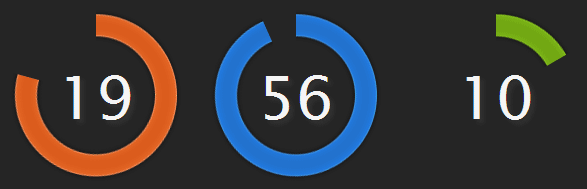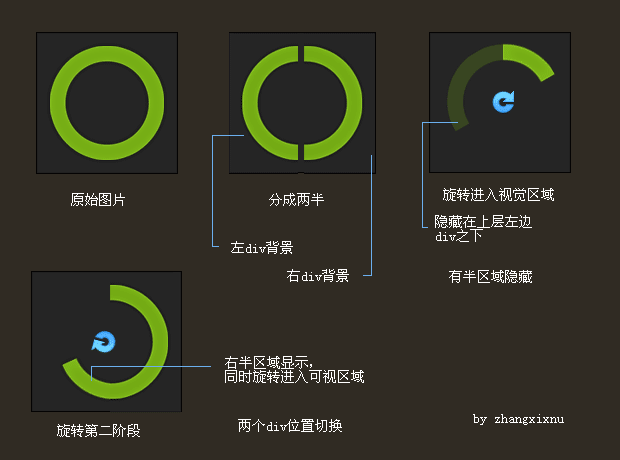## CSS3+js实现多彩炫酷旋转圆环时钟效果### 二、实现原理简介`-moz-transform:rotate(-90deg); -webkit-transform:rotate(-90deg);`

IE浏览器是使用其私有的滤镜，类似下面的写法：

`filter:progid:DXImageTransform.Microsoft.Matrix(M11=cos(roation),M12=-sin(roation),M21=sin(roation),M22=cos(roation),SizingMethod='auto expand');`

```obj.style.filter = "progid:DXImageTransform.Microsoft.Matrix(M11=0.5,M12=-0.866,M21=0.866,M22=0.5,SizingMethod='auto expand')";
obj.style.MozTransform = l.style.WebkitTransform = l.style.transform = "rotate(60deg)";
```

`var offsety = offsetx = (200 - 200 * Math.sqrt(2) * Math.cos(Math.PI / 180 * Math.abs(angle - 45))) / 2 ;`

### 三、结语

（本篇完）

﻿
1. 小bug说道：

11:60:00

2. sky说道：

您好，我下载案例 看了一点 ie10及ie10以下都不兼容哈，怎么破~~

3. EOC说道：

牛！~~~~

4. 无意中发现楼主的空间说道：